# Overview

This article will give an explanation of the different discounting engines in Cloud Retailer.

# Procedure

## Discount Types

Product Discounts and Custom Discounts are the same, except Product Discounts are applied automatically when matching products are added to a transaction at the POS. Custom Discounts must be explicitly applied via a Task Pad button, or some other manual or automatic mechanism.

The "Create New Discount" screen is the same for both types of discounts, except Product Discounts do not have the Description field.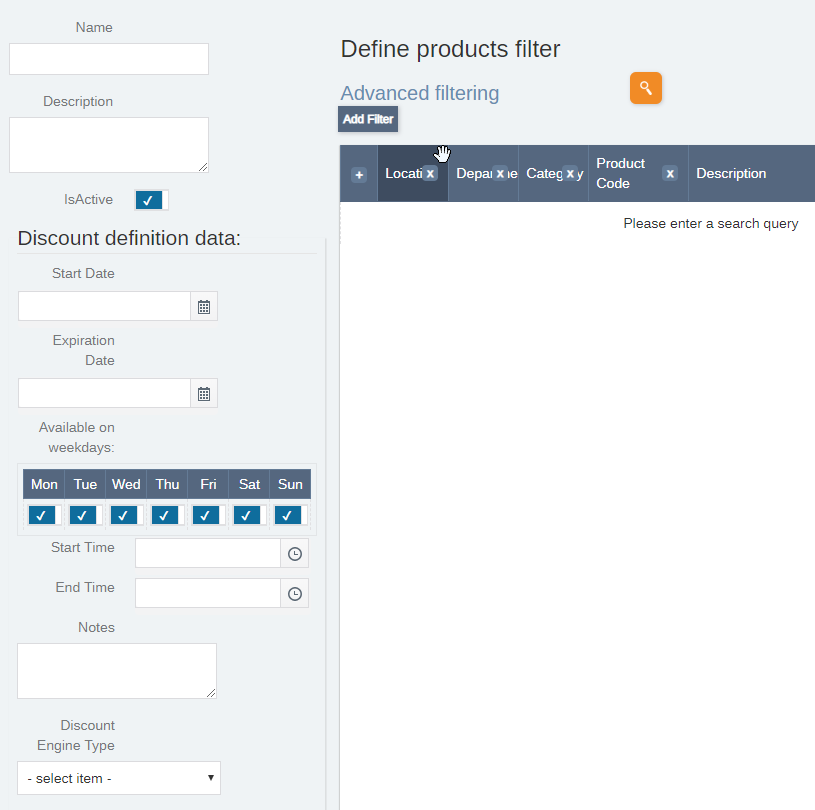### Define products filter

Add 1 or more filters to select which products are affected by the discount. For example, "Department equals Wine" AND "Category contains White".

### Discount Definition Data

• Start Date / Expiration Date: These fields define the date range within which the discount is active.
• Available on weekdays: The discount is only active on the days of the week selected.
• Start Time / End Time: These fields define the time of day during which the discount is active every day. For example, "Happy Hour".
• Discount Engine Type: This field is only active when the discount is first created. It cannot be changed after the discount is saved for the first time. The Discount Engine Types are explained below.

## Discount Engine Types

### Percent Off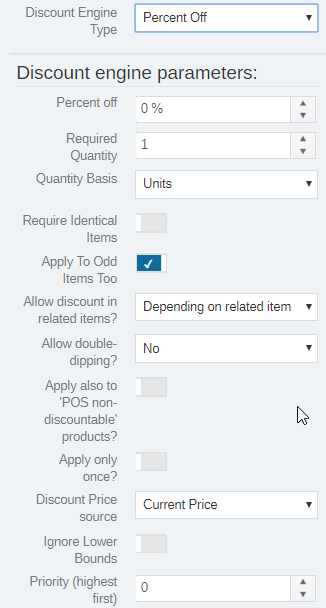This discount gives a percentage off the price of the line item when the Required Quantity is reached.
• Percent off: The percentage discount taken from the Discount Price source.
• Required Quantity: The minimum number of units that must be purchased for the discount to be active.
• Quantity Basis:
• Units: The discount is applied when the number of product units reaches the Required Quantity (e.g.; 12 bottles of wine for a case discount).
• Size: The Required Quantity is the total of the container sizes of the matching products (e.g.; 9000 ml of wine for a case discount). The container size is defined in the Size definition that has been applied to the product.
• Require Identical Items:
• No: Will only apply a discount if all matching products in all matching line items have a combined quantity higher than the required quantity.
• Same Product: Will only apply a discount if all matching line items with the same product have a combined quantity higher than the required quantity
• Same Line Item:  Will only apply a discount to the single line item if the required quantity is higher than the required quantity.
• Apply To Odd Items Too: If this option is checked then any additional items past the Required Quantity receive the discount (e.g.; with a buy 3 and get 10% off sale, the 4th item also gets 10% off). If this option is not checked, then any additional items past the Required Quantity do not receive the discount until a multiple of the Required Quantity is reached again. (e.g.; with a buy 3 and get 10% off sale, the 4th and 5th items do not qualify for the 10% off until there is a 6th item).
• Allow discount in related items?:
• Depending on related item:
• Always:
• Never:
• Allow double-dipping?:
• Never (not even with strong double-dipped): Never allow this discount if another discount has been applied to this item.
• No: Never allow this discount if another discount has been applied to this item, unless the other discount is set to Strong double-dipping.
• Regular (only with other double-dipped discounts): Allow this discount if there are other discounts of type Regular or Strong already applied to this line item.
• Strong (also with non double-dipped discounts): Always allow this discount when there are other discounts applied to this line item.
• Apply also to 'POS non-discountable' products?: If this option is checked, then the discount can be applied even if the product has the option Do not allow discounts at POS enabled in its product definition screen.
• Apply only once?:
• Discount Price source: Select which price level is used as the basis for calculating this discount (e.g.; setting this to Regular Price will always use the product's regular price to calculate the discount, even if the product is currently on sale).
• Ignore Lower Bounds: If this option is enabled, the discounted price can be lower than the Low bounds price defined in the product's definition screen.
• Priority (highest first): This number defines the order in which discounts are applied if multiple discounts can apply to the same product. The highest numbers are applied first.
• PLEASE NOTE SOME OF THE SETTINGS ABOVE APPLY TO ALL DISCOUNT ENGINE TYPES.

### Fixed Price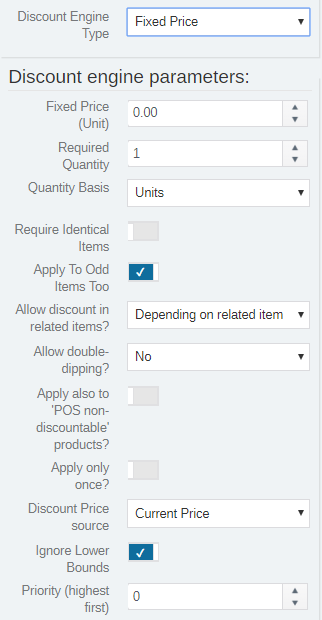This discount sets the unit price of the line items to a set amount when the Required Quantity is reached.

• Fixed Price (Unit): This is the price for a single unit of the product after the Required Quantity is reached.
It is possible to enter a decimal value to the nth decimal place. In doing so, you will need to adjust the "Discount rounding scale".
• Discount rounding scale: This is set to 2 decimal places by default.
If you choose to use a more precise fixed price, this value would need to match how my decimal places are used.
Example: I have a fixed price of 0.595238. I am using a precision of six decimal places. My discount rounding scale would equal six.

### Buy Some - Get Some CheaperThis discount applies to a number of line items after the Buy Amount is reached.

• Buy Amount: This is the number of items that must be bought before the discount goes into effect. These items will not receive a discount.
• Get Amount: This is the number of items that will receive the discount.
• Percent off: This is the percentage discount applied to the Get Amount items. If this value is zero, then the Unit Price value will be used instead.
• Unit price (if percent off = 0): This is the discount unit price that will be applied to the Get Amount items if the Percent off value is zero.

### Price Level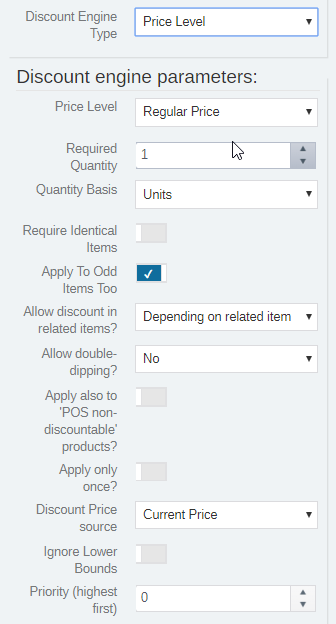This discount sets the price level of line items after the Required Quantity is reached.

• Price Level: This is the price level the line items will use after the Required Quantity is reached.

### Cost Plus PercentThis discount sets the price of line items to a percentage over the product's cost after the Required Quantity is reached.

• Price Level: This is the percent (profit margin) that will be added to the product cost to calculate the discount price after the Required Quantity is reached.

### Fixed Total DiscountThis discount sets the extended price of each applicable line item to the value of Fixed Total, no matter what the quantity of that line item is.

• Fixed Total: This value sets the extended price of the line item.
It is possible to enter a decimal value to the nth decimal place. In doing so, you will need to adjust the "Discount rounding scale".
• Discount rounding scale: This is set to 2 decimal places by default.
If you choose to use a more precise fixed price, this value would need to match how my decimal places are used.
Example: I have a fixed price of 0.595238. I am using the precision of six decimal places. My discount rounding scale would equal to six.

### Dollar Off Each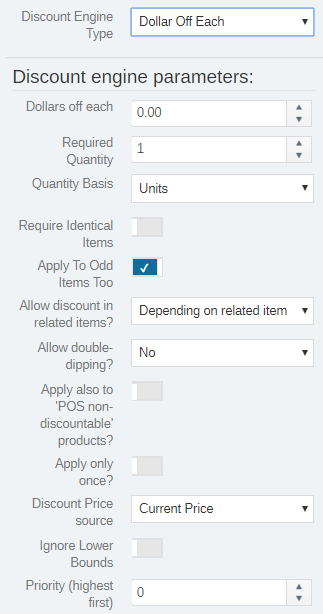This discount will apply a fixed dollar amount discount to the unit price of each line item if the Required Quantity is reached.

• Dollars off each: This is the amount that will be discounted from the unit price of each applicable line item after the Required Quantity is reached.

### Dollar Off - One TimeThis discount subtracts a fixed amount from the extended price of a line item.

• Dollar off: This value sets the discount to the extended price of the line item.

### Product Setting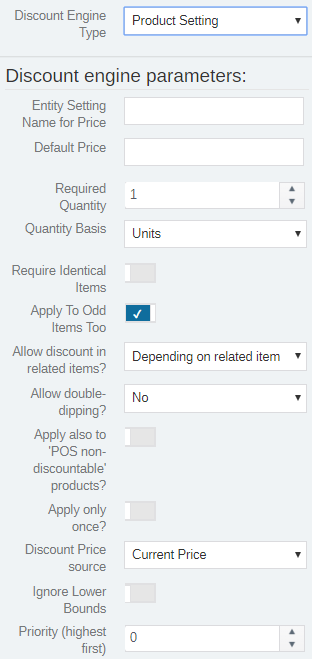This discount is similar to the Price Level discount, but it uses the value set in a Product Entity Setting for the unit price.

• Entity Setting Name for Price: The name of the Product Entity Setting that holds the unit price value.
• Default price: The unit price to use if the Entity Setting Name for Price is not found.

### Composite DiscountThis discount will apply multiple other discounts at once to the matching line items.

• Discount definitions: This is a comma-separated list of discount names.

NOTE:  After you create a discount, be sure you exit out of the POS and go back in before attempting to use it.